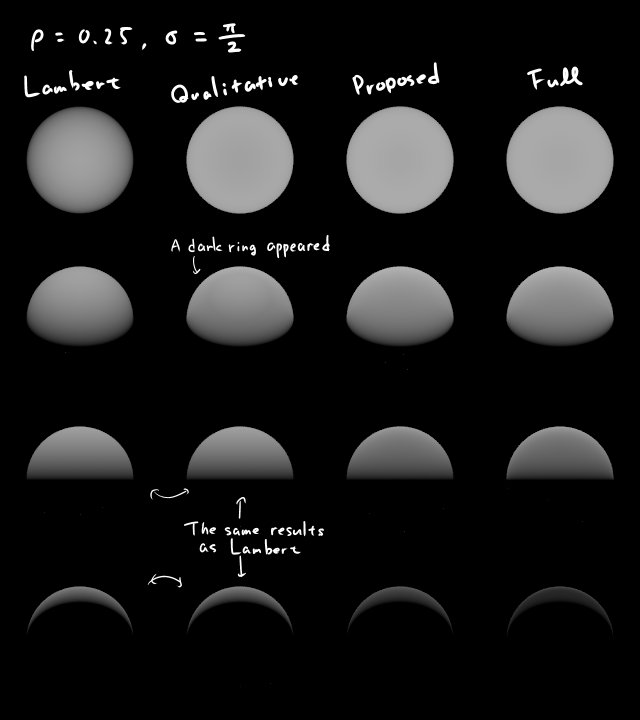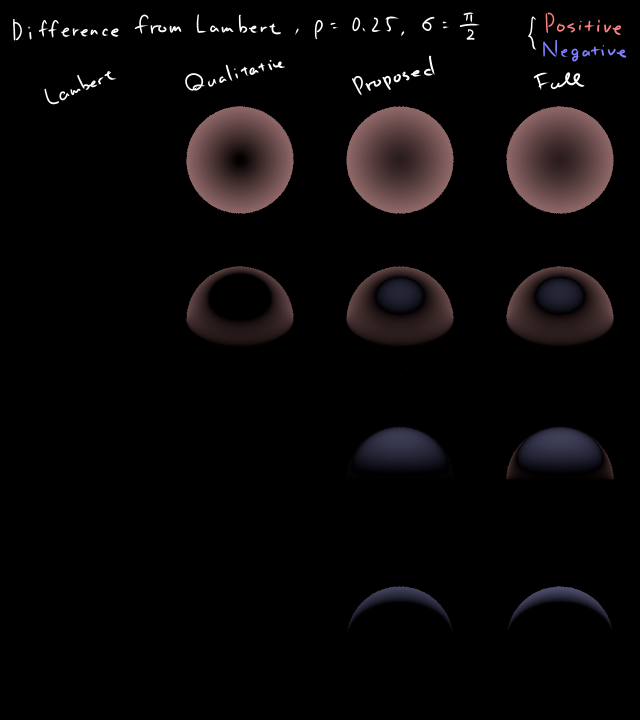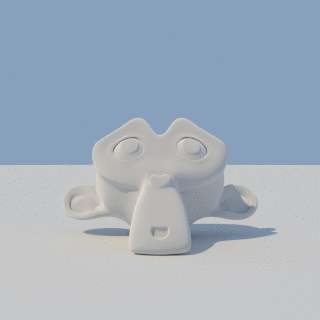$\newcommand{\block}{\begin{#1} #2 \end{#1}} \newcommand{\D}{\mathrm{d}} \renewcommand{$$}{\left(} \renewcommand{$$}{\right)}$

# A tiny improvement of Oren-Nayar reflectance model

## Introduction

M. Oren and S. K. Nayar have proposed a reflectance model of rough diffuse surfaces and two approximate functions (We call them "full O-N" and "qualitative O-N" respectively in this article) in 1993 [link] [link] . Full O-N approximate the model very well, but it is complex and has many computationally-expensive functions. Qualitative O-N, which is widely used in CG community, is simple but it has some problems. For example:

• Sometimes undesired dark rings are observed on spheres [img]. These are sometimes highly visible in animated scenes with moving lights.
• Fall back to the Lambert model when $$L \cdot V \le 0$$.

I introduce a slightly modified version of the qualitative O-N. It features:

• Simple.
• The complexity is the same as qualitative O-N. No sin, cos, arccos, nor sqrt are required. It contains only 3 inner products and 1 scalar division.
• Very similar result as full O-N.

## Notation

\block{align*}{ \vec{L} &= (\theta_i, \phi_i) &: &\text{ light source direction} \\ \vec{V} &= (\theta_r, \phi_r) &: &\text{ viewing direction} \\ \vec{N} & &: &\text{ surface normal} }
\block{align*}{ \phi &:= \phi_r - \phi_i }

We use θ to denote polar angles and φ to denote azimuth angles on the surfaces.

## Proposed formula

Using a vector notation, proposed formula is described as follows.

\block{align*}{ s &= L \cdot V - (N \cdot L) (N \cdot V) \\ t &= \block{cases}{ 1 & \text{if } s \le 0 \\ \max(N \cdot L, N \cdot V) & \textrm{if } s \gt 0 } }
\block{align*}{ L_\text{iON}(N, L, V) &= \rho \ (N \cdot L) $$A + B \frac{s}{t}$$ }

A and B are the constant numbers which depend on the roughness of the surfaces. Following formula can be used to match the result with full O-N:

\block{align*}{ A &= \frac{1}{\pi} \left( 1 - 0.5 \frac{\sigma^2}{\sigma^2 + 0.33} + 0.17 \rho \frac{\sigma^2}{\sigma^2 + 0.13} \right) \\ B &= \frac{1}{\pi} \left( 0.45 \frac{\sigma^2}{\sigma^2 + 0.09} \right) }

It violates energy conservation law, $$\int \D \omega_i L \le 1$$ when $$\rho \gt 0.97$$. However, full O-N also has this problem.

Proposed formula simply consists of a linear combination of the diffuse term and the non-diffuse term, so I propose a artificial, but useful parameterization of σ.

\block{align*}{ 0 \le \sigma' \le 1 }
\block{align*}{ A &= \frac{1}{\pi + \left( \frac{\pi}{2} - \frac{2}{3} \right) \sigma'} \\ B &= \frac{\sigma'}{\pi + \left( \frac{\pi}{2} - \frac{2}{3} \right) \sigma'} }

where σ' has a simple meaning: mixing ratio of (non-diffuse term) / (diffuse term). Normalization factor is determined to keep overall intensity at $$V \cdot N = 0$$, which is a maximal point of non-diffuse term, therefore it never violates energy conservation law.

## Result

Comparison between full O-N (blue), qualitative O-N (green) and proposed (red) at $$\rho = 0.8, \sigma = \pi / 4$$.

 00.svg 10.svg 20.svg 01.svg 11.svg 21.svg 02.svg 12.svg 22.svg

The worst case is $$\theta_i \simeq \frac{\pi}{2} \wedge \theta_r \simeq \frac{\pi}{2} \wedge \phi \simeq \frac{\pi}{2}$$. But suppose that the directions of the surfaces are uniformly distributed in a scene, the area of each surface on the screen is proportional to $$\cos \theta_i \cos \theta_r$$. So the worst case occupies relatively small region.

## Analysis

We can evaluate $$\cos \phi$$ by projecting N and V on the surface.

\block{align*}{ \cos \phi &= \frac{L - (N \cdot L) N}{\left| L - (N \cdot L) N \right|} \cdot \frac{V - (N \cdot V) N}{\left| V - (N \cdot V) N \right|} \\ &= \frac{L \cdot V - (N \cdot L) (N \cdot V)}{\sin \theta_i \sin \theta_r} }

Therefore, proposed formula is described in spherical coordinates as follows:

\block{align*}{ s &= \cos \phi \sin \theta_i \sin \theta_r \\ t^{-1} &= \block{cases}{ 1 & \text{if } s \le 0 \\ 1 / \max( \cos \theta_r, \cos \theta_i ) & \text{if } s \gt 0 } }
\block{align*}{ L_\text{iON}(\theta_r, \theta_i, \phi) &= \rho \cos \theta_i (A + B s t^{-1}) }

On the other hand, qualitative O-N can be written as the following form:

\block{align*}{ s &= \cos \phi \sin \theta_i \sin \theta_r \\ t^{-1} &= \block{cases}{ 0 & \text{if } s \le 0 \\ 1 / \max( \cos \theta_r, \cos \theta_i ) & \text{if } s \gt 0 } }
\block{align*}{ L_\text{qON}(\theta_r, \theta_i, \phi) &= \rho \cos \theta_i (A' + B' s t^{-1}) }

As you see, proposed formula is very similar to qualitative O-N.

Dark rings on spheres are appeared on the borders of $$s = 0$$. Qualitative O-N has 1st-order discontinuity at $$\theta_r = 0$$, wheareas proposed formula has 3rd-order discontinuity. Dark rings are completely disappeared on proposed formula.

s is always non-positive when $$L \cdot V \le 0$$ (see the definition of s in vector notation), hence qualitative O-N falls back to Lambertian even if $$\sigma \ne 0$$, whereas proposed formula doesn't.

$$L_\text{iON}$$ satisfy the following physical requirements.

\block{align*}{ L_\text{iON}(\theta_r, \theta_i, \phi) \cos \theta_r &= L_\text{iON}(\theta_i, \theta_r, \phi) \cos \theta_i && \text{(Helmholtz reciprocity)} \\ L_\text{iON}(\theta_r, \theta_i, \phi ; \sigma = 0) &= \frac{\rho}{\pi} \cos \theta_i && \text{(Equivalent to Lambertian when  \sigma = 0 )} \\ \int_0^{2 \pi} \D \phi \int_0^{\frac{\pi}{2}} \D \theta_i \sin \theta_i &{} L_\text{iON}(\theta_r, \theta_i, \phi) \le 1 && \text{(Energy conservation)} }

Moreover, when the domains of $$(\theta, \phi)$$ are extended to negative values, $$L_\text{iON}( \theta_r, \theta_i, \phi )$$ satisfy the following equalities without any modification.

\block{align*}{ L_\text{iON}(\theta_r, \theta_i, \phi) &= L_\text{iON}(\theta_r, \theta_i, -\phi) \\ L_\text{iON}(-\theta_r, \theta_i, \phi) &= L_\text{iON}(\theta_r, \theta_i, \phi \pm \pi) \\ L_\text{iON}(\theta_r, -\theta_i, \phi) &= L_\text{iON}(\theta_r, \theta_i, \phi \pm \pi) }

It may imply that the combinations of trigonometric functions used in this formula seem to be natural.

## Rendered images

I implemented proposed formula to Blender cycles.Comparison between qualitative O-N and proposed formula at $$A = B \ ( \sigma' = 1.0 )$$ .cd ..

Yasuhiro Fujii <y-fujii at mimosa-pudica.net>